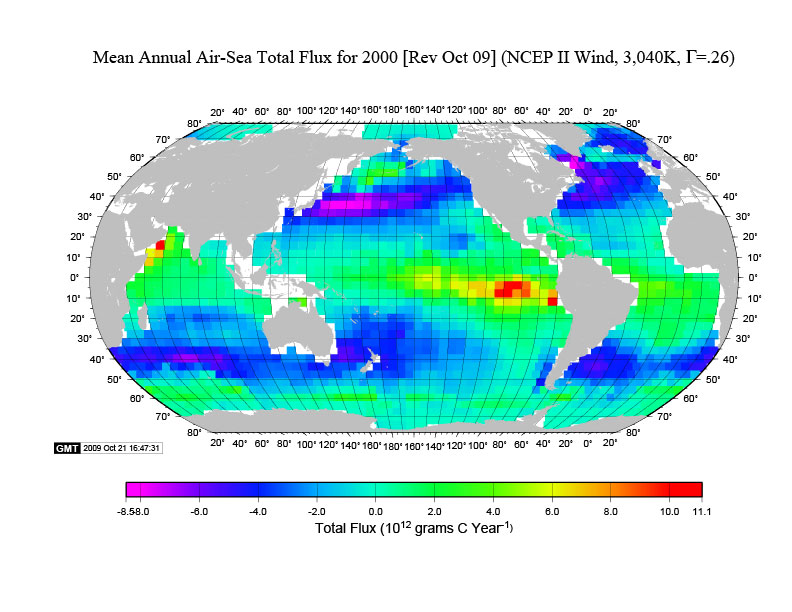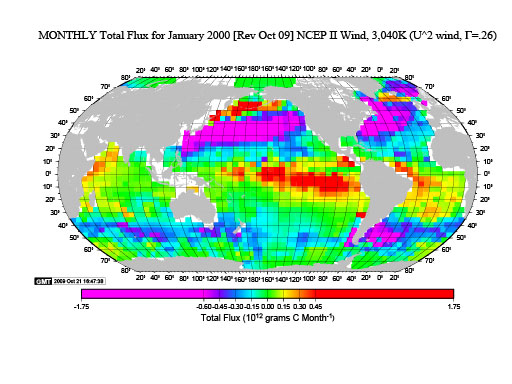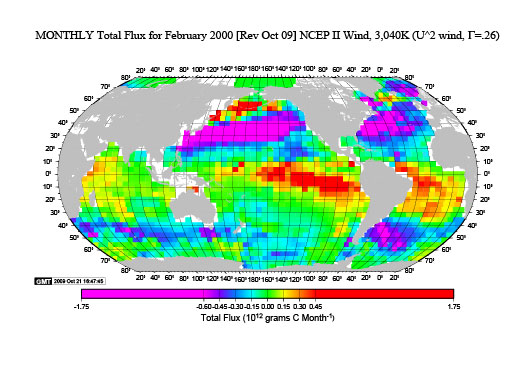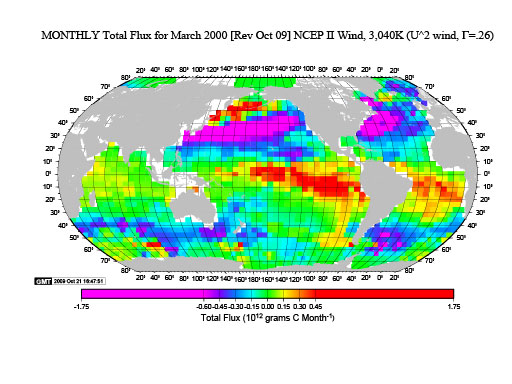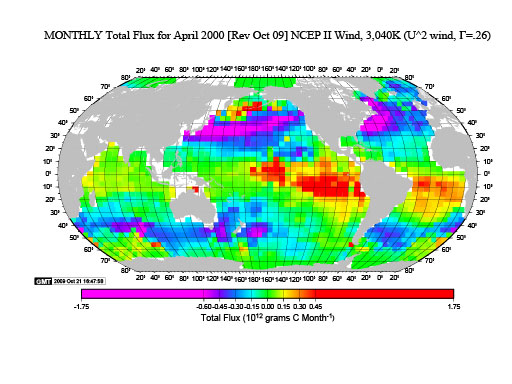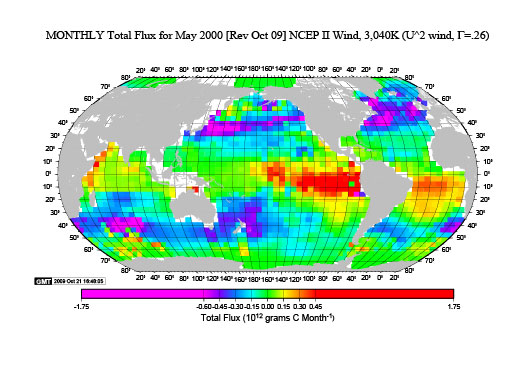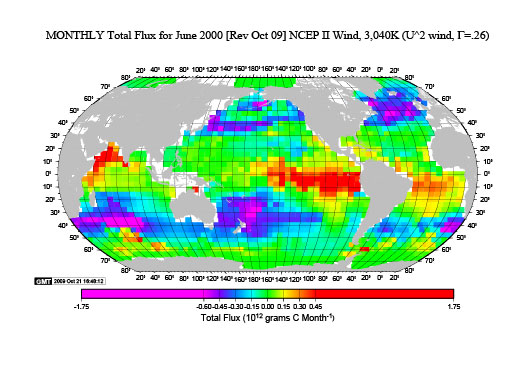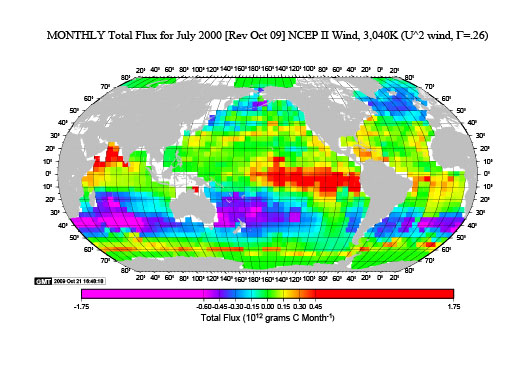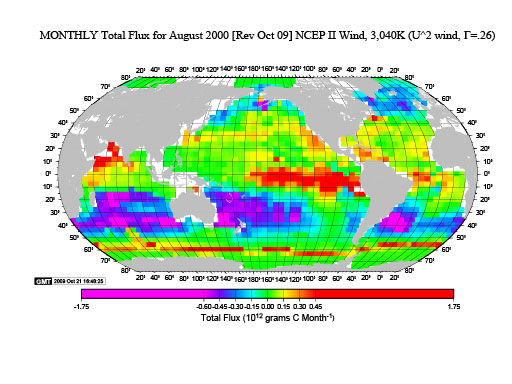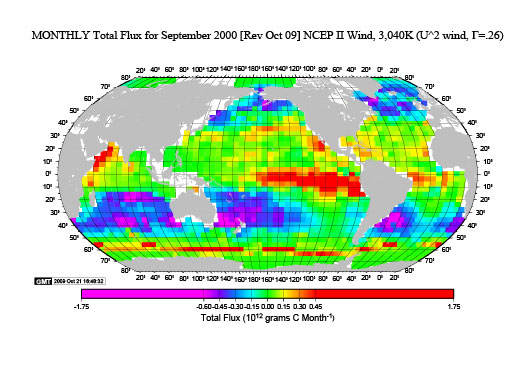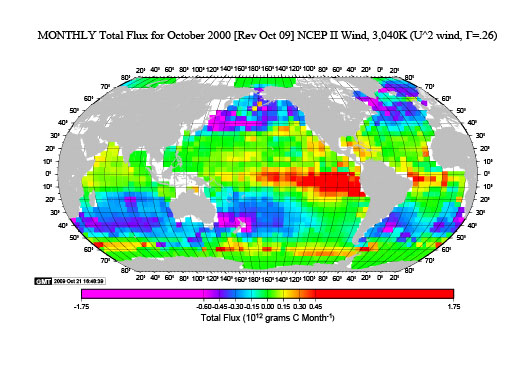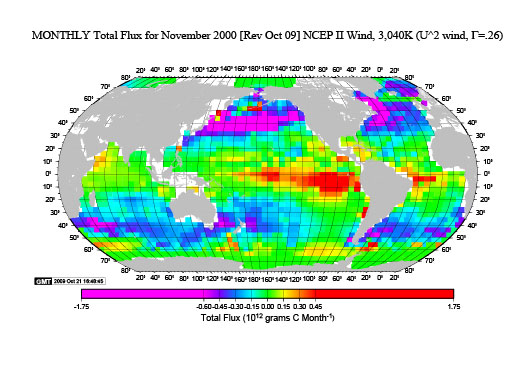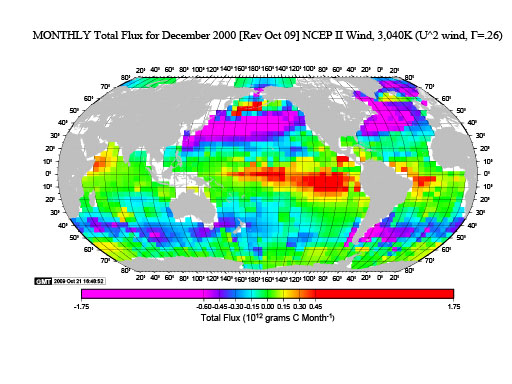These maps show the total Carbon flux from each 4° latitude x 5° longitude box for each month or each year. For the annual map, the unit is 10^12 grams Carbon per year from each box. For the monthly maps the unit is 10^12 grams Carbon per month from each box. Essentially this is the flux per unit area multiplied by the area of each box. (Revised October 2009).